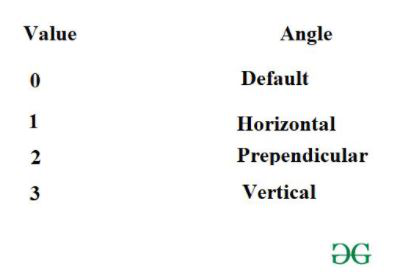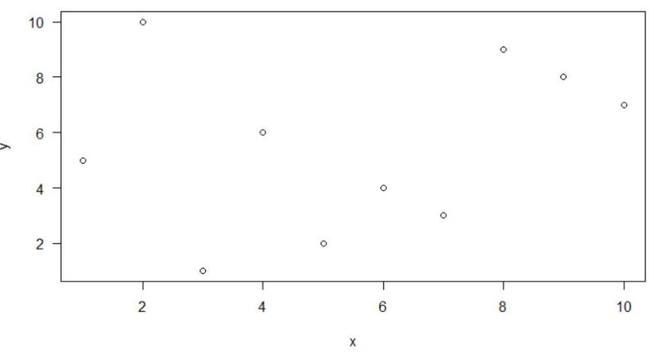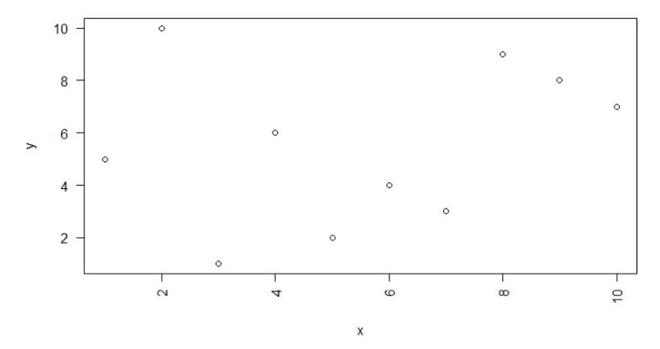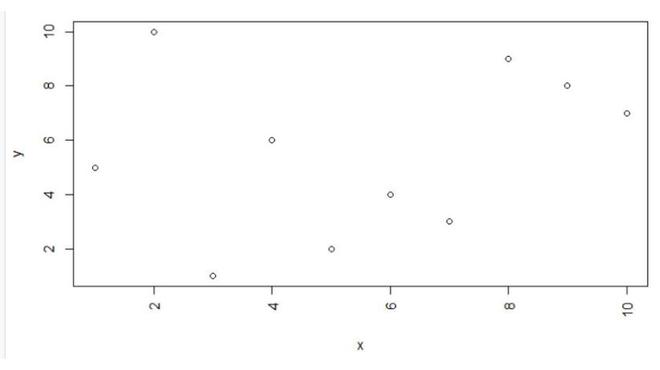# Rotate Axis Labels of Base R Plot

• Last Updated : 31 Aug, 2021

In this article, we are going to rotate the axis labels of the plot in the R programming language.

To do this the user just needs to call the base function plot and use the las argument of this function with the specific values into it and this will be leading to the rotation of the labels of the plot as per the las argument value passed in the R programming language.

#### Values of the las argument as per the rotation angle:Plot function: This is a generic function for plotting R objects

Syntax: plot(x, y,las …)

Parameters:

• x: the x coordinates of points in the plot
• y: the y coordinates of points in the plot
• las – A numeric value indicating the orientation of the tick mark labels and any other text added to a plot after its initialization

## Rotate axis labels horizontally

In this example, we will be rotating the axis labels of the base R plot of 10 data points to the horizontal position by the use of the plot function with the las argument with its value as 1 in the R programming language.

## R

 `x = ``c``(2, 7, 9, 1, 4, 3, 5, 6, 8, 10) ` `y = ``c``(10, 3, 8, 5, 6, 1, 2, 4, 9, 7) ` `plot``(x, y, las=1) `

Output:## Rotate axis labels perpendicular to the axis

In this example, we will be rotating the axis labels of the base R plot of 10 data points same as used in the previous example to the perpendicular position by the use of the plot function with the las argument with its value as 2 in the R programming language.

## R

 `x = ``c``(2, 7, 9, 1, 4, 3, 5, 6, 8, 10) ` `y = ``c``(10, 3, 8, 5, 6, 1, 2, 4, 9, 7) ` `plot``(x, y, las=2) `

Output:## Rotate axis labels vertical to the axis

In this example, we will be rotating the axis labels of the base R plot of 10 data points same as used in the previous example to the vertical position by the use of the plot function with the las argument with its value as 3 in the R programming language.

## R

 `x = ``c``(2, 7, 9, 1, 4, 3, 5, 6, 8, 10) ` `y = ``c``(10, 3, 8, 5, 6, 1, 2, 4, 9, 7) ` `plot``(x, y, las=3) `

Output:My Personal Notes arrow_drop_up
Recommended Articles
Page :